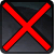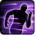# [Nitro]Conditions

## Related effects, buffs and debuffs

• [3s][Nitro]

 Slot: Debuff Duration: 3 Sek. Tick rate: does not tick # occurrences: 1
• On Apply

Only when the following conditions are met:

Perform the following actions:

• Set movement speed to 50%
• Custom
- Param 4 = (string)
- Param 5 = (string)
- Param 6 = (string)
- Param 7 = (string)
- Param 8 = (string)
- Param 9 = (string)
- Param 1 = (int) 9399188432865711031
- Param 3 = (int) 0
- Param 2 = (float) 60
• Play appearance epp . world_design . generic . explosion . fan_explosion
• On Apply

Only when the following conditions are met:

• If previous subeffect did not meet all conditions

Perform the following actions:

• Set movement speed to 700%
• Custom
- Param 4 = (string)
- Param 5 = (string)
- Param 6 = (string)
- Param 7 = (string)
- Param 8 = (string)
- Param 9 = (string)
- Param 1 = (int) 9399188432865711031
- Param 3 = (int) 0
- Param 2 = (float) 80
• Just before this effect is removed

Only when the following conditions are met:

• If PRIMARY TARGET has an effect with the tag tag.​abl.​test.​joshuam.​race_prototype.​race_mount

Perform the following actions:

• Custom
- Param 4 = (string)
- Param 5 = (string)
- Param 6 = (string)
- Param 7 = (string)
- Param 8 = (string)
- Param 9 = (string)
- Param 1 = (int) 9399188432865711031
- Param 3 = (int) 0
- Param 2 = (float) 70
• Just before this effect is removed

Only when the following conditions are met:

• If previous subeffect did not meet all conditions

Perform the following actions:

• Custom
- Param 4 = (string)
- Param 5 = (string)
- Param 6 = (string)
- Param 7 = (string)
- Param 8 = (string)
- Param 9 = (string)
- Param 1 = (int) 9399188432865711031
- Param 3 = (int) 0
- Param 2 = (float) 50